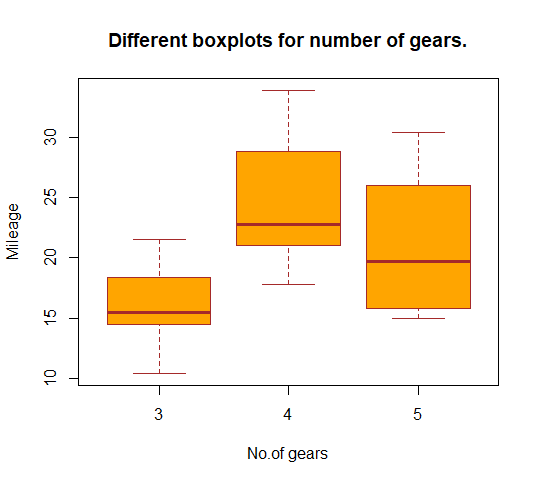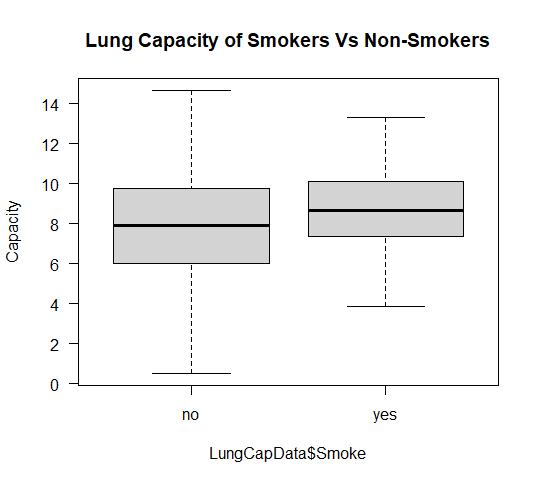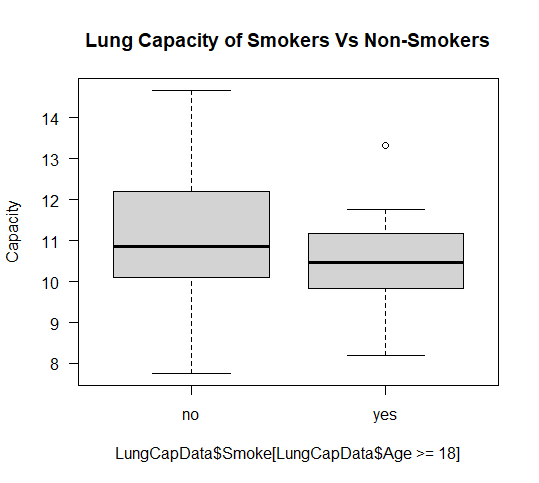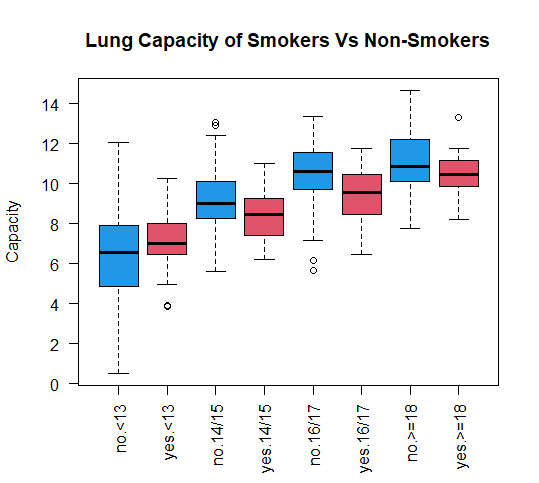Open In App

# Stratified Boxplot in R Programming

A boxplot is a graphical representation of groups of numerical data through their quartiles. Box plots are non-parametric that they display variation in samples of a statistical population without making any assumptions of the underlying statistical distribution. The spacings between the different parts of the box in a boxplot indicate the degree of dispersion and skewness in the data and show outliers. Boxplot can be drawn either vertically or horizontally. Boxplot got their name from the box in the middle. Stratified boxplots are used to examine the relationship between a categorical and a numeric variable, between strata or groups defined by a third categorical variable. Stratified Boxplots are useful when it comes to comparing categorical variables.

### Implementation in R

In R programming stratified boxplot can be formed using the boxplot() function of the R Graphics Package.

Syntax:

boxplot(formula, data = NULL, …, subset, na.action = NULL, xlab = mklab(y_var = horizontal),

ylab = mklab(y_var =!horizontal), add = FALSE, ann = !add, horizontal = FALSE, drop = FALSE,

sep = “.”, lex.order = FALSE)

boxplot(x, …, range = 1.5, width = NULL, varwidth = FALSE, notch = FALSE, outline = TRUE, names, plot = TRUE,

border = par(“fg”), col = NULL, log = “”, pars = list(boxwex = 0.8, staplewex = 0.5, outwex = 0.5),

ann = !add, horizontal = FALSE, add = FALSE, at = NULL)

Example 1:

To plot the stratified boxplot use mtcars datasets of the datasets library in R. mtcars datasets contains data from the Motor Trend Car Road Tests. Here let’s plot the mileage(miles/gallons in this case) of different cars to the number of gears they have.

## R

 `# Import the required library``library``(datasets)`` ` `# Import the dataframe``cars <- ``data.frame``(mtcars)`` ` `# Using boxplot()``boxplot``(mpg~gear, data = mtcars,``        ``main = ``"Different boxplots for number of gears."``,``        ``xlab = ``"No.of gears"``,``        ``ylab = ``"Mileage"``,``        ``col = ``"orange"``,``        ``border = ``"brown"``)`Example 2:

The dataset we are working with here is the LungCapData dataset which contains data on lung capacities of smokers and non-smokers of different age groups. The structure of the datasets has 6 variables each signifying lung capacity, age, height, smoke(‘yes’ for a smoker and ‘no’ for a non-smoker), gender(male/female), and Caesarean(yes/no) of a person. We will divide the ages into groups and then try to plot stratified boxplots for the lung capacity of smokers vs non-smokers with age strata. Please download the CSV file here.

## R

 `# Load the dataset``LungCapData <- ``read.csv``(``"LungCapData.csv"``, header = T)``LungCapData <- ``data.frame``(LungCapData)``attach``(LungCapData)`` ` `# Catgorise Age into groups``AgeGroups <- ``cut``(LungCapData\$Age,``                 ``breaks = ``c``(0, 13, 15, 17, 25),``                 ``labels = ``c``(``"<13"``, ``"14/15"``, ``"16/17"``, ``">=18"``))``head``(LungCapData)`` ` `# BoxPlot 1``boxplot``(LungCapData\$LungCap~LungCapData\$Smoke,``        ``ylab = ``"Capacity"``, ``        ``main = ``"Lung Capacity of Smokers Vs Non-Smokers"``,``        ``las = 1)`` ` `# BoxPlot 2``boxplot``(LungCapData\$LungCap[LungCapData\$Age>=18]~LungCapData\$Smoke[LungCapData\$Age>=18],``        ``ylab = ``"Capacity"``,``        ``main = ``"Lung Capacity of Smokers Vs Non-Smokers"``,``        ``las = 1)`` ` `# BoxPlot 3``boxplot``(LungCapData\$LungCap~LungCapData\$Smoke*AgeGroups,``        ``ylab = ``"Capacity"``, xlab = ``""``,``        ``main = ``"Lung Capacity of Smokers Vs Non-Smokers"``,``        ``col = ``c``(4, 2), las = 2)`

### Output:

# Boxplot 1

Boxplot 1 plots the lung capacity of smokers and non-smokers, where no symbolize non-smokers, and yes symbolizes smokers.By analyzing the above-shown boxplot we can clearly say the lung capacity of non-smokers is lower as compared to that of smokers on an average.

# Boxplot 2

Boxplot 2 plots the lung capacity of smokers and non-smokers of age group greater or equal to 18, where no symbolizes non-smokers and yes symbolizes smokers.# Boxplot 3

Boxplot 3 plots the lung capacity of smokers and non-smokers of the different age groups in the dataset where blue-colored boxplots are for non-smokers and red is for smokers.